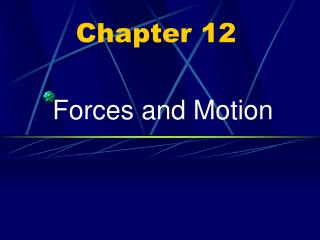DownloadDownload PresentationChapter 12

# Chapter 12

Download Presentation## Chapter 12

- - - - - - - - - - - - - - - - - - - - - - - - - - - E N D - - - - - - - - - - - - - - - - - - - - - - - - - - -
##### Presentation Transcript

1. Chapter 12 Forces and Motion

2. Force Force is a push or a pull.

3. Force A force can cause: • A resting object to move • Accelerate a moving object By changing the object's • Speed • Direction

4. Measuring Force

5. Measuring Force lbs - Pound N - Newton

6. Earth Connecting Motion and Forces Force (newton, N): A push or pull one body exerts on another.

7. Balanced Forces Balanced Forces: Forces on an object that are equal in size and opposite in direction.

8. 5 N 3 N Unbalanced Forces Unbalanced Forces: Forces on an object are not equalresulting in a Net Force.

9. 5 N 3 N Unbalanced Forces Unbalanced Forces: When an unbalanced force acts on an object, the object accelerates.

10. 2 N Net Force A Net Force on an object always changes the velocity of the object.

11. Balanced Forces Balanced Forces: When the forces on an object are balanced, the net force is zero and there is no change in the object's motion.

12. Friction Force that opposes the motion of objects that touch as they move past each other.

13. Static Friction A friction force that acts on objects that are not moving.

14. Sliding Friction A friction force that opposes the motion of an object as it slides over a surface.

15. Rolling Friction

16. Rolling Friction A friction force that acts on rolling objects, caused by the change in shape at the point of rolling contact.

17. Fluid Friction AirResistance

18. Fluid Friction

19. Fluid Friction A friction force that opposes the motion of an object through a fluid.

20. Gravity Gravity: Every object in the universe exerts a force on every other object. This force is Gravity!!!

21. Gravity Gravity causes objects to accelerate downward, whereas air acts in the direction opposite to the motion and reduces acceleration.

22. Gravity AirResistance

23. Projectile Motion

24. Projectile Motion

25. Horizontal Component Vertical Component Projectile Motion

26. Projectile Motion The combination of initial forward velocity and the downward vertical force of gravity causes the ball to follow a curved path.

27. Physical Science: 12-1 Worksheet: 12-1 Due:3/4/04

28. Aristotle Force was required to keep an object moving at a constant speed.

29. Aristotle Object will move indefinitely if no force is applied.

30. Newton

31. Newton

32. Newton

33. Inertia Inertia (mass): The tendency of an object to resist any change in its motion. 25 kg 1kg

34. The more mass an object has, the greater its inertia.

35. Newton’s First Law The Law of Inertia An object in moving tends to stay in motion at a constant velocity and an object at rest tends to remain at rest unless a net force acts on it.

36. Newton’s First Law

37. F a Newton’s Second Law of Motion The Law of Acceleration A net force acting on an object will accelerate that object in the direction of that force

38. FNet FF FE Net Force Accelerates FE - engine FF - friction FNet – net force FNet = FE -FF

39. FN FE=25N FF =5N Net Force Accelerates FN = FE-FF FN = 25N –5N FN = 20N

40. Newton’s Second Law Acceleration Force = mass x acceleration F = ma

41. Fa Fm F ma F = m= m a a=

42. Example #1 A car with a mass of 2000kg is parked on the side of the road. How much force is needed to accelerate the car at 3m/s²? (Assume that there is no friction against the car.)

43. F Example #1

44. Given: m = 2000kg a = 3m/s² Find: F = ? Equation: F = ma F = (2000kg) x(3m/s²) F = 15,000N

45. Weight Weight: The measure of the force of gravity on an object.